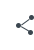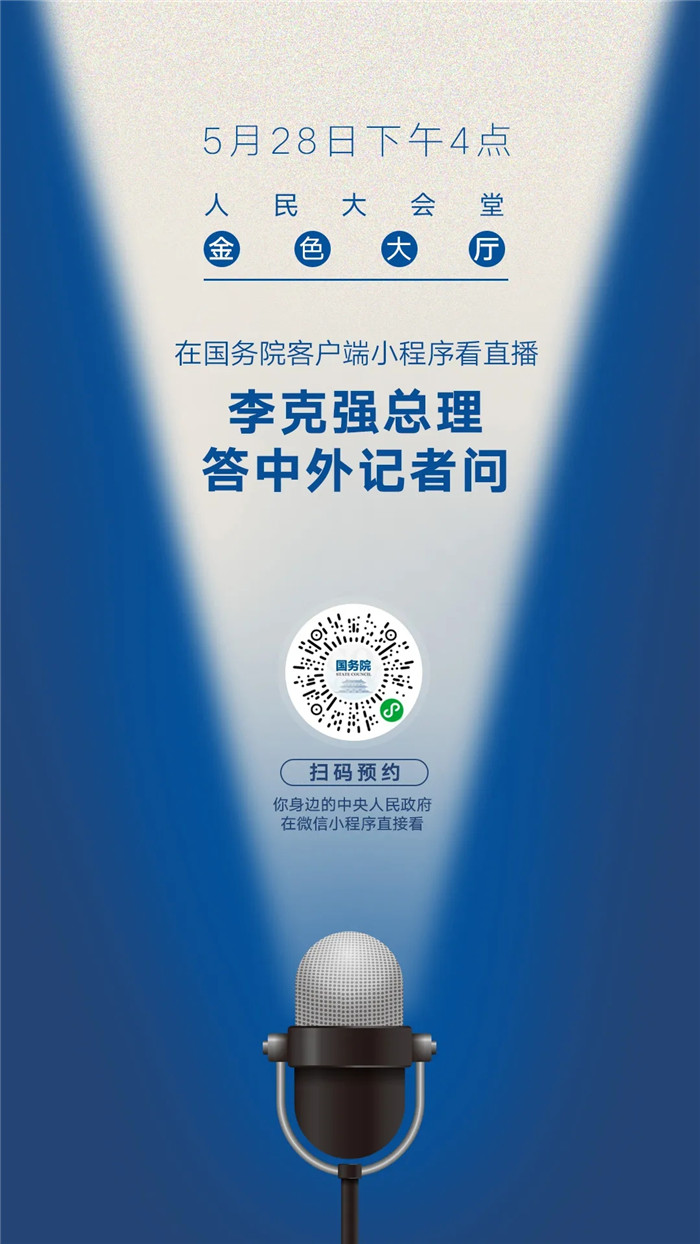# 下午4点李克强总理答中外记者问，直播见！

###### 来源：中国政府网日期：2020-05-28 视力保护色： \$(document).ready(function(){ \$(".share_box").click(function(){ \$(".share_cell").toggle(); }) })收藏 .news-sc{display: inline-block;position: relative;text-align: right!important;;} .news-sc .favbox{cursor: pointer;background:url(../../../images/icon_tofav_200706.png) center left no-repeat;padding-left: 20px;} .news-sc .favbox.susbed{background-image: url(../../../images/icon_isfav_200706.png);color: #0058a8;} .news-sc>.tips{position: absolute;top: 0;right: 55px;display: none; width: 150px;} function encodeUTF8(s) { var i, r = [], c, x; for (i = 0; i < s.length; i++) if ((c = s.charCodeAt(i)) < 0x80) r.push(c); else if (c < 0x800) r.push(0xc0 + ((c >> 6) & 0x1f), 0x80 + (c & 0x3f)); else { if ((x = c ^ 0xd800) >> 10 == 0) //对四字节UTF-16转换为Unicode (c = (x << 10) + (s.charCodeAt(++i) ^ 0xdc00) + 0x10000), r.push(0xf0 + ((c >> 18) & 0x7), 0x80 + ((c >> 12) & 0x3f)); else r.push(0xe0 + ((c >> 12) & 0xf)); r.push(0x80 + ((c >> 6) & 0x3f), 0x80 + (c & 0x3f)); } return r; } // 字符串加密成 hex 字符串 function sha1(s) { var data = new Uint8Array(encodeUTF8(s)); var i, j, t; var l = (((data.length + 8) >>> 6) << 4) + 16, s = new Uint8Array(l << 2); s.set(new Uint8Array(data.buffer)), (s = new Uint32Array(s.buffer)); for (t = new DataView(s.buffer), i = 0; i < l; i++) s[i] = t.getUint32(i << 2); s[data.length >> 2] |= 0x80 << (24 - (data.length & 3) * 8); s[l - 1] = data.length << 3; var w = [], f = [ function () { return (m & m) | (~m & m); }, function () { return m ^ m ^ m; }, function () { return (m & m) | (m & m) | (m & m); }, function () { return m ^ m ^ m; }, ], rol = function (n, c) { return (n << c) | (n >>> (32 - c)); }, k = [1518500249, 1859775393, -1894007588, -899497514], m = [1732584193, -271733879, null, null, -1009589776]; (m = ~m), (m = ~m); for (i = 0; i < s.length; i += 16) { var o = m.slice(0); for (j = 0; j < 80; j++) (w[j] = j < 16 ? s[i + j] : rol(w[j - 3] ^ w[j - 8] ^ w[j - 14] ^ w[j - 16], 1)), (t = (rol(m, 5) + f[(j / 20) | 0]() + m + w[j] + k[(j / 20) | 0]) | 0), (m = rol(m, 30)), m.pop(), m.unshift(t); for (j = 0; j < 5; j++) m[j] = (m[j] + o[j]) | 0; } t = new DataView(new Uint32Array(m).buffer); for (var i = 0; i < 5; i++) m[i] = t.getUint32(i << 2); var hex = Array.prototype.map .call(new Uint8Array(new Uint32Array(m).buffer), function (e) { return (e < 16 ? "0" : "") + e.toString(16); }) .join(""); return hex; } function RndNum(n) { var rnd = ""; for (var i = 0; i < n; i++) rnd += Math.floor(Math.random() * 10); return rnd; } jQuery.support.cors = true; jQuery.ajaxSetup({ xhr: function () { if (window.ActiveXObject) { return new window.ActiveXObject("Microsoft.XMLHTTP"); } else { return new window.XMLHttpRequest(); } }, }); var guidecollectionid = ""; var userName = ""; \$(function () { var iframe = document.getElementById("gjdl"); if (iframe.attachEvent) { iframe.attachEvent("onload", function () { getGuideCollection(); }); } else { iframe.onload = function () { getGuideCollection(); }; } //点击星星 var userId = userInfo.userId; var apiHost = "https://szfkhd.jilinxiangyun.com"; var regionCode = "220600"; \$(".favbox").on("click", function () { var userId = userInfo.userId; var taskCode = \$("#wdid").val(); //唯一值 var tit = \$("meta[name='ArticleTitle']").attr("content"); var timestamp = Math.round(new Date().getTime() / 1000).toString(); //时间戳 var nonce = RndNum(10); //随机10位数 var newsAbstract = \$("meta[name='ColumnDescription']").attr("content"); var newsUrl = window.location.href; var newsImgUrl = ""; var newsReleaseTime = \$("meta[name='PubDate']").attr("content"); var newsReleaseDepartment = \$("meta[name='SiteName']").attr("content"); var collectSource = 2; var appId = "pc_ciYSMiROK"; var keyy = "irMH0IjWBrX8X4UJ"; var region = regionCode; var array = [ appId, keyy, timestamp, nonce, userId, taskCode, collectSource, region, ]; array = array.sort(); var token = ""; for (var i = 0; i < array.length; i++) { token += array[i]; } token = sha1(token); if (islogin) { console.log(\$(this).hasClass("susbed")); if (\$(this).hasClass("susbed")) { //已收藏进行删除收藏 \$(".favbox").attr("disabled", true); \$.ajax({ url: apiHost + "/api/v1/news/cancelCollect", type: "post", headers: { device: "4", }, data: { appId: appId, timestamp: timestamp, nonce: nonce, token: token, userId: userId, newsId: taskCode, collectSource: 2, region: region, }, dataType: "json", success: function (data) { console.log(data); \$(".favbox").attr("disabled", false); if (data.code == "200") { //如果实心图标 点击后变成空心 \$(".news-sc .tips").text("收藏取消"); \$(".news-sc .tips").show().delay(1000).fadeOut(); \$(".icon_fav").removeClass("susbed"); } }, }); } else { var userId = userInfo.userId; var taskCode = \$("#wdid").val(); //唯一值 var tit = \$("meta[name='ArticleTitle']").attr("content"); var timestamp = Math.round(new Date().getTime() / 1000).toString(); //时间戳 var nonce = RndNum(10); //随机10位数 var newsAbstract = \$("meta[name='ColumnDescription']").attr("content"); var newsUrl = window.location.href; var newsImgUrl = ""; var newsReleaseTime = \$("meta[name='PubDate']").attr("content"); var newsReleaseDepartment = \$("meta[name='SiteName']").attr("content"); var collectSource = 2; var appId = "pc_ciYSMiROK"; var keyy = "irMH0IjWBrX8X4UJ"; var region = regionCode; var array = [ appId, keyy, timestamp, nonce, userId, taskCode, tit, newsUrl, collectSource, region, ]; array = array.sort(); var token = ""; for (var i = 0; i < array.length; i++) { token += array[i]; } token = sha1(token); \$.ajax({ url: apiHost + "/api/v1/news/addCollect", headers: { device: "4", }, type: "post", data: { appId: appId, timestamp: timestamp, nonce: nonce, token: token, userId: userId, newsId: taskCode, newsTitle: tit, newsUrl: newsUrl, newsImgUrl: "", collectSource: 2, region: region, }, dataType: "json", success: function (data) { console.log(data); \$(".favbox").attr("disabled", false); if (data.code == "200") { //如果空心图标 点击后变成实心 //\$fbc.addClass("shoucang_i_v") \$(".news-sc .tips").text("收藏成功"); \$(".news-sc .tips").show().delay(1000).fadeOut(); \$(".icon_fav").addClass("susbed"); } }, }); } } else { unLoginDefaultHandleFn(); } }); getGuideCollection(); //判断是否收藏过 function getGuideCollection() { if (islogin) { var userId = userInfo.userId; var taskCode = \$("#wdid").val(); //唯一值 var tit = \$("meta[name='ArticleTitle']").attr("content"); var timestamp = Math.round(new Date().getTime() / 1000).toString(); //时间戳 var nonce = RndNum(10); //随机10位数 var newsAbstract = \$("meta[name='ColumnDescription']").attr("content"); var newsUrl = window.location.href; var newsImgUrl = ""; var newsReleaseTime = \$("meta[name='PubDate']").attr("content"); var newsReleaseDepartment = \$("meta[name='SiteName']").attr("content"); var collectSource = 2; var appId = "pc_ciYSMiROK"; var keyy = "irMH0IjWBrX8X4UJ"; var region = regionCode; var array = [ appId, keyy, timestamp, nonce, userId, taskCode, collectSource, region, ]; array = array.sort(); var token = ""; for (var i = 0; i < array.length; i++) { token += array[i]; } token = sha1(token); \$.ajax({ url: apiHost + "/api/v1/news/collectStatus?appId=" + appId + "&timestamp=" + timestamp + "&nonce=" + nonce + "&token=" + token + "&userId=" + userId + "&newsId=" + taskCode + "&collectSource=" + collectSource + "&region=" + region + "", type: "get", dataType: "json", headers: { device: "4", }, success: function (data) { console.log(data); if (data != "无") { if (data.data.status == 1) { guidecollectionid = data.data.keyid; \$(".news-sc .tips").text("收藏成功"); \$(".icon_fav").addClass("susbed"); //收藏过变成实心星星 } else { \$(".news-sc .tips").text("收藏取消"); \$(".icon_fav").removeClass("susbed"); //空心星星 } } }, error: function (XMLHttpRequest, textStatus, errorThrown) { // 状态码 console.log(XMLHttpRequest.status); // 状态 console.log(XMLHttpRequest.readyState); // 错误信息 console.log(textStatus); }, }); } } });